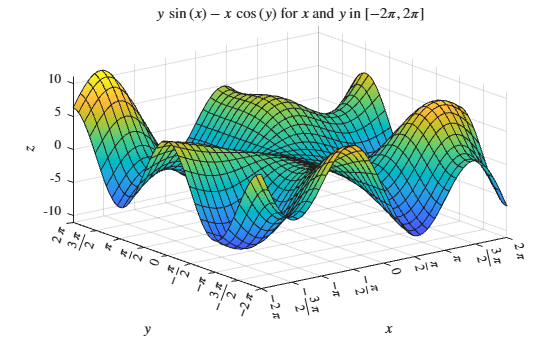# latex

LaTeX form of symbolic expression

## Syntax

chr = latex(S)

## Description

example

chr = latex(S) returns the LaTeX form of the symbolic expression S.

## Examples

collapse all

Find the LaTeX form of the symbolic expressions x^2 + 1/x and sin(pi*x) + phi.

syms x phi chr = latex(x^2 + 1/x)
chr = '\frac{1}{x}+x^2' 
chr = latex(sin(pi*x) + phi)
chr = '\phi +\sin\left(\pi \,x\right)' 

Find the LaTeX form of the symbolic array S.

syms x S = [sym(1)/3 x; exp(x) x^2]
S =  $\left(\begin{array}{cc}\frac{1}{3}& x\\ {\mathrm{e}}^{x}& {x}^{2}\end{array}\right)$
chr = latex(S)
chr = '\left(\begin{array}{cc} \frac{1}{3} & x\\ {\mathrm{e}}^x & x^2 \end{array}\right)' 

Perform computation using several symbolic matrix variables, and then find their LaTeX forms.

Create 3-by-3 and 3-by-1 symbolic matrix variables.

syms A 3 matrix syms X [3 1] matrix

Find the Hessian matrix of ${X}^{T}AX$. Derived equations involving symbolic matrix variables appear in typeset as they would be in textbooks.

f = X.'*A*X
f = ${X}^{\mathrm{T}} A X$
H = diff(f,X,X.')
H = ${A}^{\mathrm{T}}+A$

Generate the LaTeX forms of the symbolic matrix variables f and H.

chrf = latex(f)
chrf = '{\textbf{X}}^{\mathrm{T}}\,\textbf{A}\,\textbf{X}' 
chrH = latex(H)
chrH = '{\textbf{A}}^{\mathrm{T}}+\textbf{A}' 

Perform computation using symbolic matrix functions, and then find their LaTeX forms.

Create a 3-by-1 symbolic matrix variable.

syms X [3 1] matrix

Create a symbolic matrix function that represents the formula $\mathit{f}\left(\mathbit{X}\right)={\mathbit{X}}^{\mathit{T}}\mathbit{X}$.

syms f(X) [1 1] matrix keepargs f(X) = X.'*X
f(X) = ${X}^{\mathrm{T}} X$

Find the derivative of $\mathit{f}\left(\mathbit{X}\right)$ with respect to $\mathbit{X}$.

Df = diff(f,X)
Df(X) = $2 {X}^{\mathrm{T}}$

Evaluate the derivative for the matrix value $\mathbit{X}=\left[1;\text{\hspace{0.17em}}2;\text{\hspace{0.17em}}3\right]$.

DfEval = Df([1; 2; 3])
DfEval =  

Generate the LaTeX forms of the symbolic matrix functions f and Df, and the symbolic expression DfEval.

chrf = latex(f)
chrf = '{\textbf{X}}^{\mathrm{T}}\,\textbf{X}' 
chrDf = latex(Df)
chrDf = '2\,{\textbf{X}}^{\mathrm{T}}' 
chrEval = latex(DfEval)
chrEval = '2\,{\left(\begin{array}{c} 1\\ 2\\ 3 \end{array}\right)}^{\mathrm{T}}' 

Modify generated LaTeX by setting symbolic preferences using the sympref function.

Generate the LaTeX form of the expression $\pi$ with the default symbolic preference.

sympref('default'); chr = latex(sym(pi))
chr = '\pi ' 

Set the 'FloatingPointOutput' preference to true to return symbolic output in floating-point format. Generate the LaTeX form of $\pi$ in floating-point format.

sympref('FloatingPointOutput',true); chr = latex(sym(pi))
chr = '3.1416' 

Now change the output order of a symbolic polynomial. Create a symbolic polynomial and set the 'PolynomialDisplayStyle' preference to 'ascend'. Generate the LaTeX form of the polynomial sorted in ascending order.

syms x; poly = x^2 - 2*x + 1; sympref('PolynomialDisplayStyle','ascend'); chr = latex(poly)
chr = '1-2\,x+x^2' 

The preferences you set using sympref persist through your current and future MATLAB® sessions. Restore the default values by specifying the 'default' option.

sympref('default');

For $x$ and $y$ from $-2\pi$ to $2\pi$, plot the 3-D surface $y\mathrm{sin}\left(x\right)-x\mathrm{cos}\left(y\right)$. Store the axes object in a by using gca. Display the axes box by using a.Box and set the tick label interpreter to latex.

Create the $x$-axis ticks by spanning the $x$-axis limits at intervals of pi/2. Convert the axis limits to precise multiples of pi/2 using round and get the symbolic tick values in S. Display the ticks by setting the XTick property of a to S. Create the LaTeX labels for the $x$-axis by using arrayfun to apply latex to S and then concatenating $. Display the labels by assigning them to the XTickLabel property of a. Repeat these steps for the $y$-axis. Set the $x$- and $y$-axes labels and the title using the latex interpreter. syms x y f = y.*sin(x)-x.*cos(y); fsurf(f,[-2*pi 2*pi]) a = gca; a.TickLabelInterpreter = 'latex'; a.Box = 'on'; a.BoxStyle = 'full'; S = sym(a.XLim(1):pi/2:a.XLim(2)); S = sym(round(vpa(S/pi*2))*pi/2); a.XTick = double(S); a.XTickLabel = strcat('$',arrayfun(@latex, S, 'UniformOutput', false),'$'); S = sym(a.YLim(1):pi/2:a.YLim(2)); S = sym(round(vpa(S/pi*2))*pi/2); a.YTick = double(S); a.YTickLabel = strcat('$',arrayfun(@latex, S, 'UniformOutput', false),'$'); xlabel('x','Interpreter','latex'); ylabel('y','Interpreter','latex'); zlabel('z','Interpreter','latex'); title(['$' latex(f) '$for$x$and$y$in$[-2\pi,2\pi]\$'],'Interpreter','latex')## Input Arguments

collapse all

Input, specified as a symbolic number, variable, vector, array, function, expression, matrix variable, or symbolic matrix function.

Data Types: sym | symfun | symmatrix | symfunmatrix

## Version History

Introduced before R2006a

expand all